#Function Repository Resource:

# AreaBetweenCurvesIntegral (1.0.0)current version: 2.0.0 »

Compute the integral describing the area between two plane curves

Contributed by: Wolfram|Alpha Math Team
 ResourceFunction["AreaBetweenCurvesIntegral"][{f,g},{x,xmin,xmax}] returns an Inactive integral representing the area of the enclosed region between the functions f(x) and g(x) over the interval xmin

## Details and Options

ResourceFunction["AreaBetweenCurvesIntegral"] works with real‐valued functions over the Cartesian coordinate system.
The area between f(x) and g(x) is defined as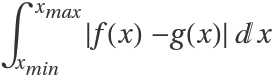.
When f(x)g(x), the area between the two curves is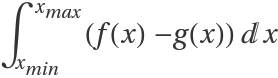.
When f(x) and g(x) only meet at x=xmin and x=xmax, the area is taken to be that of the enclosed region.
When f(x) and g(x) do not meet at x=xmin or x=xmax, the boundary of the enclosed region will contain vertical line segments joining the curves.
When f(x) and g(x) intersect for some xmin<x<xmax, the area will be that of multiple enclosed regions.
The following option can be given:
 Assumptions \$Assumptions assumptions on parameters

## Examples

### Basic Examples (3)

Compute an integral representing the area between two curves:

 In:=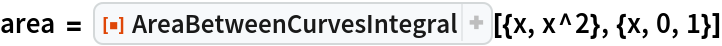Out=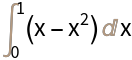Plot the region:

 In:=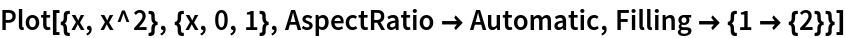Out=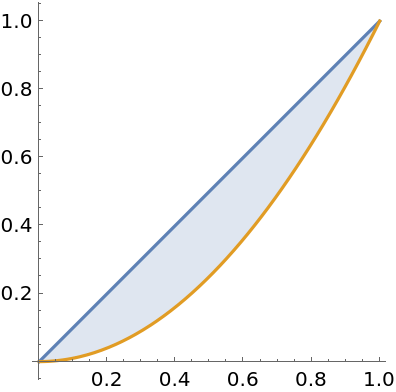Activate the integral to compute the area:

 In:=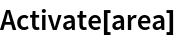Out=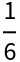### Scope (4)

Find the area of the region enclosed by two curves:

 In:=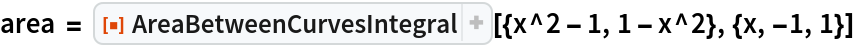Out=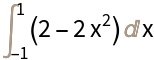In:=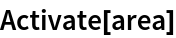Out=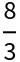Plot the region in question:

 In:=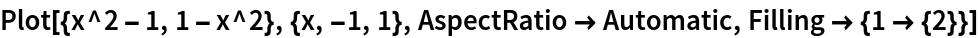Out=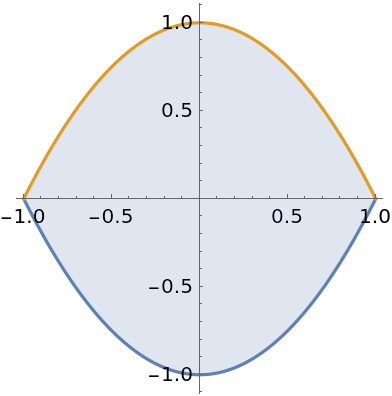A region where the curves do not meet:

 In:=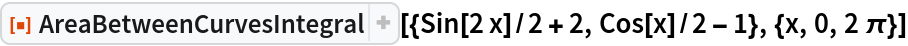Out=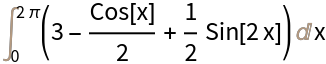In:=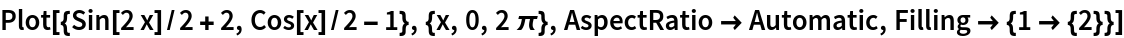Out=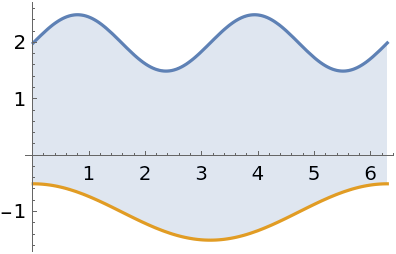With multiple enclosed regions, the integrand will use Abs to return a positive area:

 In:=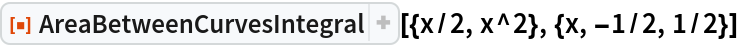Out=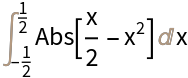Visualize the regions:

 In:=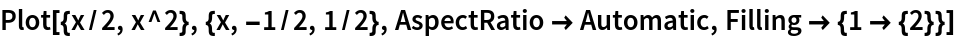Out=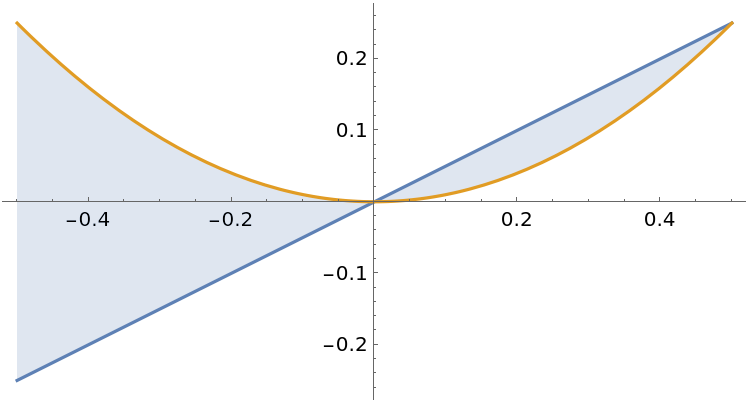Find the area between two curves containing parameters:

 In:=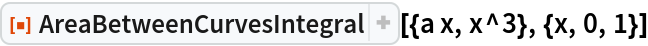Out=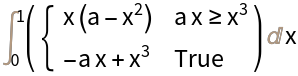### Options (2)

#### Assumptions

The result may be conditioned on parameters:

 In:=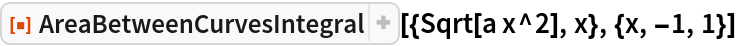Out=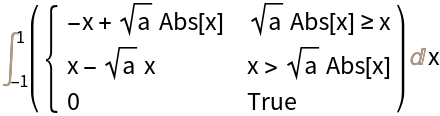Make an assumption about the parameter to then evaluate the area:

 In:=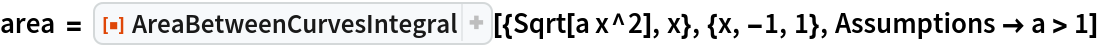Out=In:=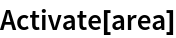Out=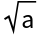### Applications (2)

Compute the area of a disk:

 In:=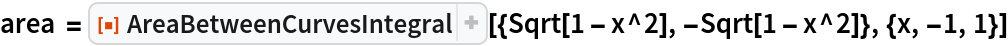Out=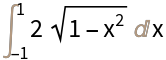In:=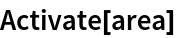Out=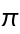Visualize the disk:

 In:=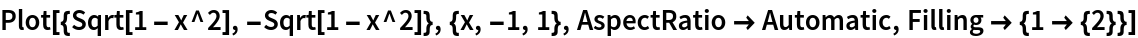Out=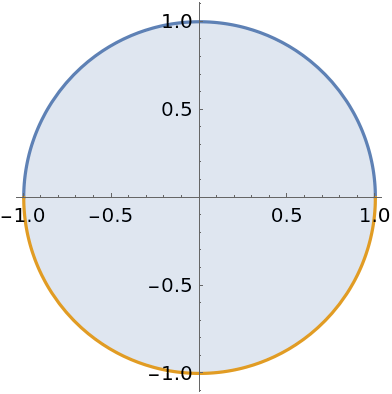Cavalieri's principle states that the area between two curves does not change when each curve is shifted by the same amount. Here are three functions:

 In:=Compute an integral representing the area between f and g:

 In:=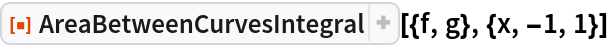Out=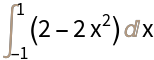This is the same as the integral obtained after shifting both f and g by h:

 In:=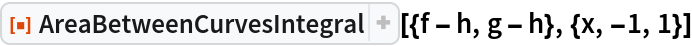Out=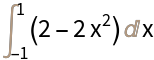Though their areas are the same, the regions are very different:

 In:=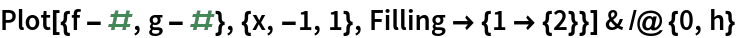Out=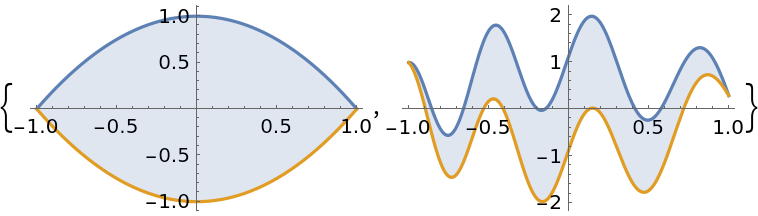### Properties and Relations (3)

Find the integral representing the area between sin(x) and cos(x) over the interval (0,π):

 In:=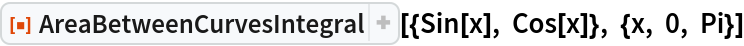Out=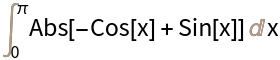Activate the integral to compute the area:

 In:=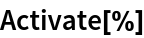Out=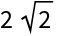Use the resource function AreaBetweenCurves to compute the area directly:

 In:=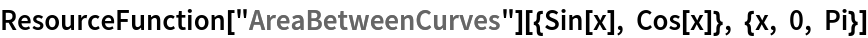Out=## Publisher

Wolfram|Alpha Math Team

## Version History

• 2.0.0 – 23 March 2023
• 1.0.0 – 11 May 2020

## Author Notes

To view underlying source code, evaluate the following:

 In:=